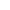# slope intercept formBest Answer pan.gejt , 02 February 2020 - 05:03 PM

I see 3 solutions. Let's suppose the line in following form: y = k *x + q

1. using direct computing, inconvenient for repeating calculations

k = (y2 - y1)/(x2 - x1)

q = y1 - k * x1 or q = y2 - k * x2

k = (-1-(-3))/(2-(-1)) = (-1 + 3)/(2 + 1) = 2/3

q = -3 - 2/3 * (-1) = -3 + 2/3 = (-9 + 2)/3 = -7/3

the equation is: y = 2/3 * x - 7/3

--------------------------------------------------------------------

2. Using linear regression

Switch your calc to Statistics menu (menu - statistics) and input x-values to List 1 and y-values to List 2 (If you have in List 1 and List 2 any data, use another number of the List - e.g. List 3 and List 4, but donť mix x-values with y-values)

Press F6, command SET and assigned lists, where you stored the data, to 2Var XList and 2Var YList.

Set 2Var Freq as 1.

Press exit, press F3 (command REG), F1 (command X), F1 (command ax+b and you will see the results:

a = 0.66666666

b = -2.3333333

the equation is: y = 2/3 * x - 7/3

My tip: If you switch your calc to Run - Matrix mode, you can write the decimal number as a fraction.

Press Vars, F3 (STAT), F3 (GRAPH) and F1 for a and F2 for b and hit enter. The values of a or b are displayed. Press the S-D to see the decimal number as the fraction.

--------------------------------------------------------------------

3. Write a small program, suitable for repeating calculations

"X1="?->A:"Y1="?->B:"X2="?->C:"Y2="?->D:

(D-B)/(C-A)->K:B-K*A->Q:

ClrText

Blue Locate 1,2,"Slope is"▲

Blue Locate 10,2,K▲

Green Locate 1,3,"ICPT is"▲

Green Locate 9,3,Q▲

Go to the full post### #1procode200

procode200

Newbie

•• Validating
•• 13 posts
• Gender:Not Telling

Posted 01 February 2020 - 07:22 PM

Is it possible to do a problem like this on CASIO fx-CG50:

Find the Equation of a Line in Slope Intercept Form Given Two Points

e.g. Find the equation (in terms of x) of the line through the points (-1,-3) and (2,-1)

### #2pan.gejt

pan.gejt

Casio Freak

•• Members
•• 253 posts
• Gender:Male
• Location:CZ

• Calculators:
.

Posted 02 February 2020 - 05:03 PM   Best Answer

I see 3 solutions. Let's suppose the line in following form: y = k *x + q

1. using direct computing, inconvenient for repeating calculations

k = (y2 - y1)/(x2 - x1)

q = y1 - k * x1 or q = y2 - k * x2

k = (-1-(-3))/(2-(-1)) = (-1 + 3)/(2 + 1) = 2/3

q = -3 - 2/3 * (-1) = -3 + 2/3 = (-9 + 2)/3 = -7/3

the equation is: y = 2/3 * x - 7/3

--------------------------------------------------------------------

2. Using linear regression

Switch your calc to Statistics menu (menu - statistics) and input x-values to List 1 and y-values to List 2 (If you have in List 1 and List 2 any data, use another number of the List - e.g. List 3 and List 4, but donť mix x-values with y-values)

Press F6, command SET and assigned lists, where you stored the data, to 2Var XList and 2Var YList.

Set 2Var Freq as 1.

Press exit, press F3 (command REG), F1 (command X), F1 (command ax+b and you will see the results:

a = 0.66666666

b = -2.3333333

the equation is: y = 2/3 * x - 7/3

My tip: If you switch your calc to Run - Matrix mode, you can write the decimal number as a fraction.

Press Vars, F3 (STAT), F3 (GRAPH) and F1 for a and F2 for b and hit enter. The values of a or b are displayed. Press the S-D to see the decimal number as the fraction.

--------------------------------------------------------------------

3. Write a small program, suitable for repeating calculations

"X1="?->A:"Y1="?->B:"X2="?->C:"Y2="?->D:

(D-B)/(C-A)->K:B-K*A->Q:

ClrText

Blue Locate 1,2,"Slope is"▲

Blue Locate 10,2,K▲

Green Locate 1,3,"ICPT is"▲

Green Locate 9,3,Q▲

Edited by pan.gejt, 02 February 2020 - 05:07 PM.

• procode200 likes this

#### 0 user(s) are reading this topic

0 members, 0 guests, 0 anonymous users